# Matching T Attenuator Calculator

Calculates the resistor values, attenuation, minimum attenuation, impedance, reflection coefficient, VSWR and return loss of an impedance matching T attenuator. This can be built into a FLEXI-BOX and a transmission line (50 Ohm track) PCB is available which easily adapts for this circuit with two simple trimming operations. There is also a selection of 50 Ohm RF connectors available.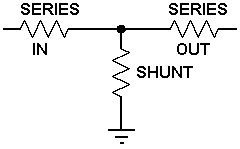1) Enter the required input and output impedance and the required attenuation then press "Calculate" below to obtain the ideal resistor values.

 INPUT DATA Input Impedance: Ohms Output Impedance: Ohms Required Attenuation: dB
 IDEAL VALUES Ideal Series In resistor: Ohms Ideal Series Out resistor: Ohms Ideal Shunt resistor: Ohms Minimum attenuation to achieve impedance match: dB

2) Change the calculated resistor values in the amber text boxes below to the nearest Preferred Resistor Values or to those available then press " Calculate" below to get the resulting attenuator parameters.

 SELECTED VALUES Series In resistor: Ohms Series Out resistor: Ohms Shunt resistor: Ohms Mismatch Losses: Included (see note below)
 PARAMETERS Forward Attenuation: dB Input Impedance: Ohms Input VSWR: Input Reflection Coefficient: Input Return Loss: dB
 Reverse Attenuation: dB Output Impedance: Ohms Output VSWR: Output Reflection Coefficient: Output Return Loss: dB

This calculator uses JavaScript and will function in most modern browsers. For more information see About our calculators

The required input and output impedance are used to calculate the minimum attenuation possible for correct impedance matching with a formula from RF Design Guide, Systems, Circuits and Equations by Peter Vizmuller Published by Artech House ISBN 0-89006-754-6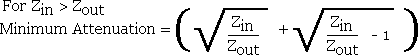Where Zin is the characteristic input system impedance

Zout is the characteristic output system impedance

Peter Vizmuller also gives formulas for calculating the resistor values directly. This calculator uses formulas that I have derived and first finds the output voltage with a source EMF of 2 Volts and therefore an input PD of 1 Volt.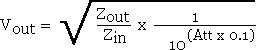The individual ideal resistor values can now be found.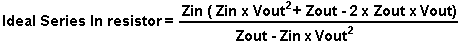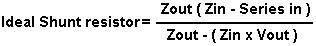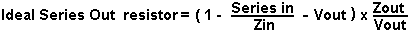After selecting preferred values, simple series and parallel resistor calculations are used to find the attenuator input impedance, input voltage and output voltage. The source EMF is again assumed to be 2 Volts.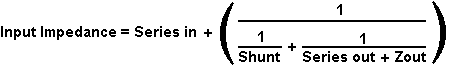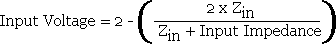The remaining formulas for Attenuation, Voltage Reflection Coefficient and VSWR are available at:-

http://www.spectrum-soft.com/news/fall2009/vswr.shtm

Note: With the Mismatch Losses box ticked the calculator will return an attenuation calculated from the source power in relation to the attenuator output power which is suitable for calculations at RF and Microwave frequencies, particularly if the attenuator is located away from the source at the end of a transmission line. Without the Mismatch Losses box ticked the calculator will return an attenuation calculated from the actual input power to the attenuator in relation to the output power which is more suitable for calculations at audio frequencies. The difference in attenuation with the two methods is only noticeable when there is a significant mismatch.